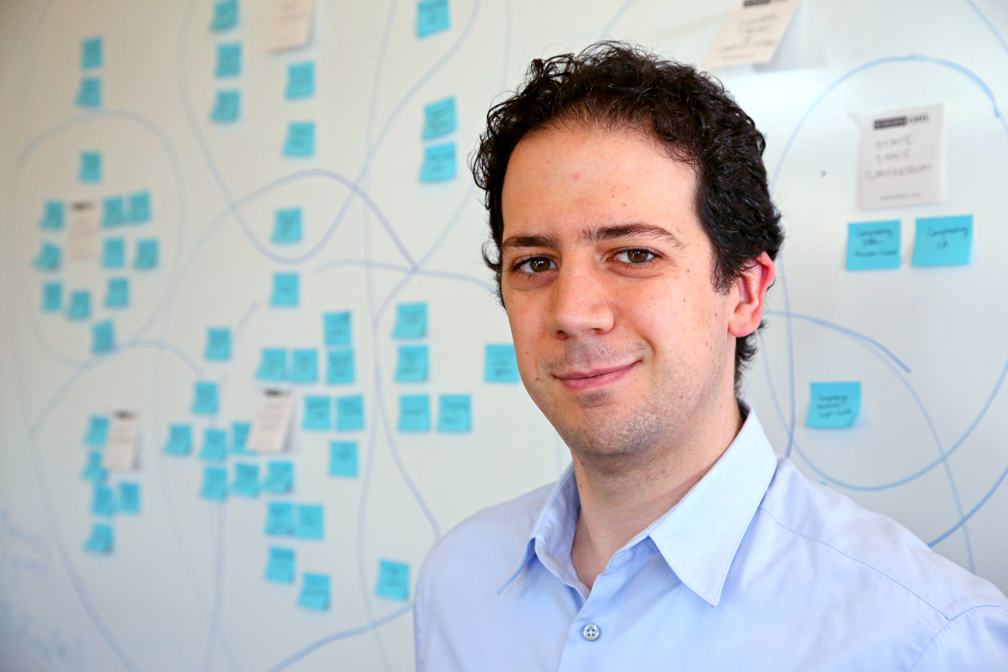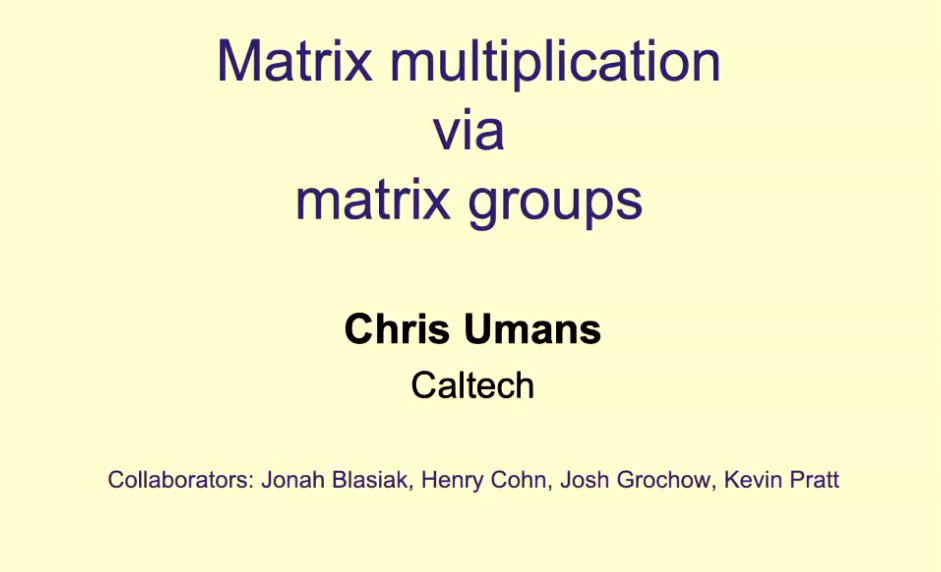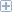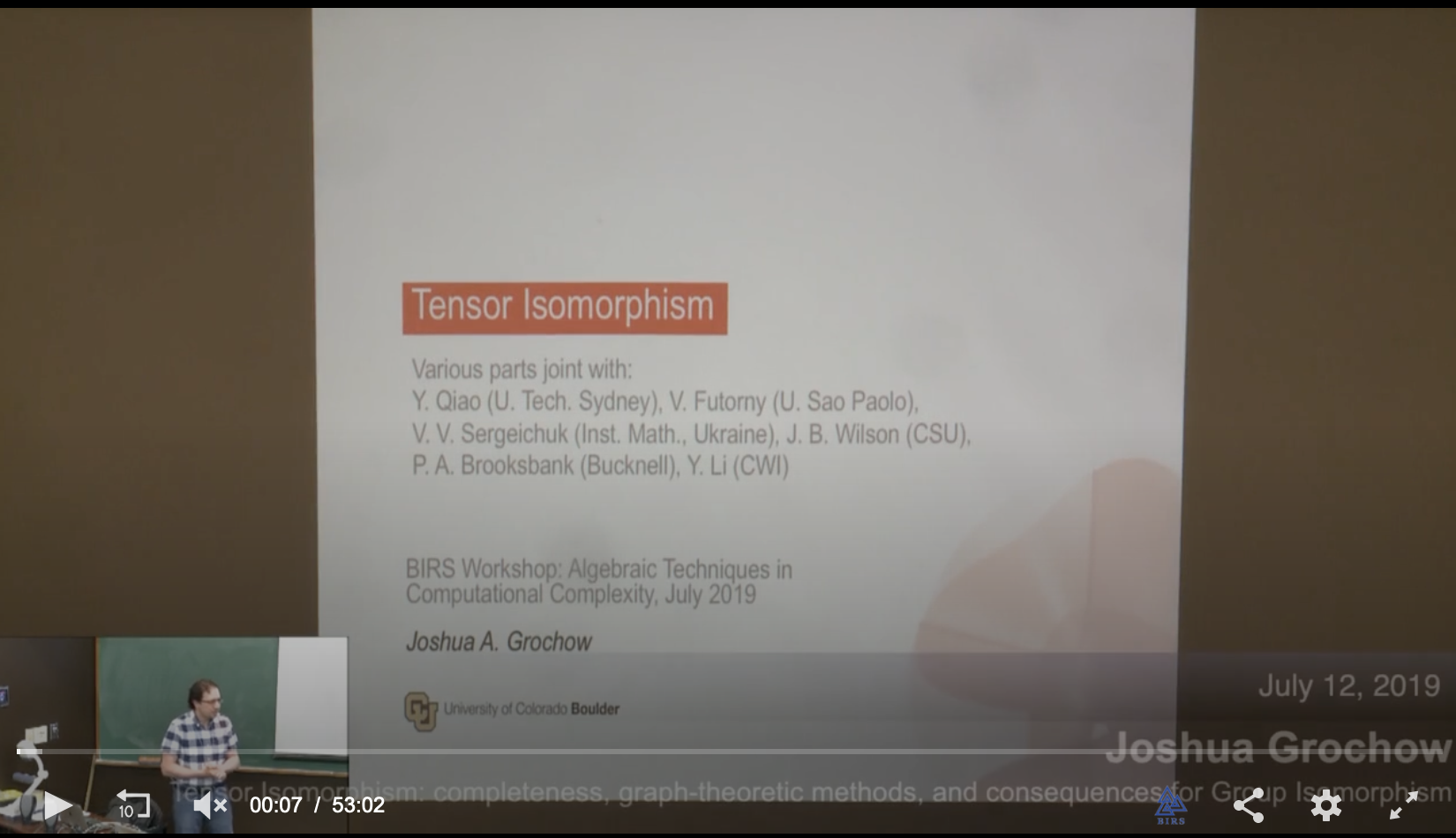Joshua A. Grochow Assistant Professor Departments of Computer Science and Mathematics University of Colorado at Boulder [first initial][last name]@colorado.edu I will not be in the office during the coronavirus pandemic. My virtual office is here on Zoom. Fax: (303) 492-2844 (ATTN: me) CS Theory @ CU Boulder Complex Systems @ CU Boulder Me @ Mastodon Twitter csthoery.SE mathoverflow ORCID My pubs @ arXiv ECCC DBLP MathSciNet Google Scholar SciRate

Hyper-matrices can be used to represent many kinds of mathematical objects, from hypergraphs and tensors, to polynomials, to groups and algebras. Over the past several years, with a variety of coauthors, we have shown that nonetheless nearly all these different settings are equivalent to one another under simple (affine) linear maps, computable in polynomial time. For example, there is a map that transforms any space of matrices into a related associative algebra in such a way that two spaces of matrices are equivalent up to change of bases if and only if the corresponding algebras are isomorphic (in the usual sense of isomorphisms of algebras). As another example, we show there is a similar map from k-tensors (equivalently, k-partite pure quantum states) to 3-tensors (tripartite states), that is, 3-tensors are sufficiently rich to simulate anything that can happen even with k-tensors. In this talk, we will discuss some of these results, the techniques that go into them, and some of their implications. Based on joint works with Futorny & Sergeichuk (Lin. Alg. Appl. 2019), and Y. Qiao (ITCS '21, J. Groups, Complexity, Cryptology, 2022 also with G. Tang, CCC '21, and upcoming preprints).Matrix multiplication via matrix groups
Presented by Chris Umans
7th Workshop on Algebraic Complexity Theory (WACT), Mar 2023
Based on our paper Matrix multiplication via matrix groups,
Joint with

Many versions of the Axiom of Choice (AC), though equivalent in ZF set theory, are inequivalent from the computational point of view. When we consider polynomial-time analogues of AC, many of these different versions can be shown to be equivalent to other more standard questions about the relationship between complexity classes. We will use some of these formulations of AC to motivate several complexity questions that might otherwise seem a bit bespoke and unrelated from one another.

Next, as many versions of AC are about cardinals, in the second half of the talk we introduce a polynomial-time version of cardinality, in the spirit of polynomial-time model theory. As this is a new theory, we will discuss some of the foundational properties of polynomial-time cardinality, some of which may be surprising when contrasted with their set-theoretic counterparts. The talk will contain many open questions, and the paper contains even more! Based on arXiv:2301.07123 [cs.CC].

Matrix multiplication via matrix groups
Presented by Kevin Pratt
14th Innovations in Theoretical Computer Science (ITCS), Jan 2023
Based on our paper Matrix multiplication via matrix groups,
Joint with

Polynomial identity testing & the ideal proof system
DIMACS Workshop on Metacomplexity, Barriers, and Derandomization, Apr 2022
Based on work in progress.

Part I: Motivation, history, and introduction to algebraic proof complexity (Toniann Pitassi)

This first talk will give a quick primer on proof complexity, especially as it relates to algebraic proof systems. It will cover some of the history and motivation that led to the study of algebraic proof systems, as well as introduce some well-studied algebraic proof systems and the relations between them.

Part II: Circuit complexity, ideals and proof systems: connections and recent results (Joshua A. Grochow)

The second talk will cover connections between the Ideal Proof System (IPS), circuit complexity, and more generally complexity in ideals. In particular, it will cover the relation between IPS and VP vs VNP, as well as the recent result of Andrews & Forbes that extends the constant-depth algebraic circuit lower bound of Limaye-Srinivasan-Tavenas to a lower bound on refuting certain polynomial equations in IPS. The talk will highlight the connection between algebraic circuit complexity, algebraic proof complexity, and even Boolean circuit complexity to the complexity not of individual (families of) polynomials, but of arbitrary (families of) polynomials inside an ideal or coset of an ideal.

On p-Group Isomorphism: search-to-decision, counting-to-decision, and nilpotency class reductions via tensors
Conference on Computational Complexity (CCC 2021), Jul 2021
Based on our paper On p-Group Isomorphism: search-to-decision, counting-to-decision, and nilpotency class reductions via tensors,
Joint with

Codes and Expansions in Algorithms for Matrix Multiplication (expository)
Codes and Expansions Seminar (CodEx), Feb 2021
Based partly on our paper Designing Strassen's algorithm,
Joint with Cris Moore.
SlidesAbstract

We overview some relationships between codes (and various code-like objects) and constructing algorithms for multiplying matrices, ranging from concrete results to intriguing observations of things that look similar if you squint just right.

This includes a connection between codes and bilinear complexity from Lempel & Winograd '77, symmetric algorithms for matrix multiplication (studied by many authors), and a construction of Strassen's 1969 matrix multiplication algorithm from a unitary 2-design (G. & Moore, arXiv:1708.09398). And more connections we won't have time for, but promise at least one slide about.

Designing Strassen's algorithm for matrix multiplication
LA Combinatorics & Complexity Seminar, Nov 2020
Based on our paper Designing Strassen's algorithm,
Joint with Cris Moore.

On the complexity of isomorphism problems for tensors, groups, and polynomials I: Tensor Isomorphism-completeness
12th Innovations in Theoretical Computer Science (ITCS), Jan 2021
Based on our paper On the complexity of isomorphism problems for tensors, groups, and polynomials I: Tensor Isomorphism-completeness,
Joint with Youming Qiao. Shorter live version with Q&A:We consider the problems of testing isomorphism of tensors, p-groups, cubic forms, algebras, and more, which arise from a variety of areas, including machine learning, group theory, and cryptography. Despite a perhaps seeming similarity with Graph Isomorphism, the current-best algorithms for these problems (when given by bases) are still exponential - for most of them, even qn2 over GF(q). Similarly, while efficient practical software exists for Graph Isomorphism, for these problems even the best current software can only handle very small instances (e.g., 10 x 10 x 10 over GF(13)). This raises the question of finding new algorithmic techniques for these problems, and/or of proving hardness results.

We show that all of these problems are equivalent under polynomial-time reductions, giving rise to a class of problems we call Tensor Isomorphism-complete (TI-complete). We further show that testing isomorphism of d-tensors for any fixed d (at least 3) is equivalent to testing isomorphism of 3-tensors. Using the same techniques, we show two first-of-their-kind results for Group Isomorphism (GpI): (a) a reduction from isomorphism of p-groups of exponent p and class c < p, to isomorphism of p-groups of exponent p and class 2, and (b) a search-to-decision reduction for the latter class of groups in time |G|O(log log|G|). We note that while p-groups of class 2 have long been believed to be the hardest cases of GpI, as far as we are aware this is the first reduction from any larger class to this class of groups. Finally, we discuss a way to apply combinatorial methods from Graph Isomorphism (namely, Weisfeiler-Leman) to Group and Tensor Isomorphism.

Based on joint works with Vyacheslav V. Futorny and Vladimir V. Sergeichuk (Lin. Alg. Appl., 2019; arXiv:1810.09219), with Peter A. Brooksbank, Yinan Li, Youming Qiao, and James B. Wilson (arXiv:1905.02518), and with Youming Qiao (arXiv:1907.00309).

Tensors & Complexity I (expository)
Tensors: Algebra-Computation-Applications (TACA), Jun 2019
Expository talk, parts of which mention key results from the following papers:
V. Futorny, J. A. Grochow, and V. V. Sergeichuk, Wildness for tensors
J. A. Grochow and Y. Qiao, On the Complexity of Isomorphism Problems for Tensors, Groups, and Polynomials I: Tensor Isomorphism-CompletenessAbstract

The first lecture will be about what computational complexity can tell us about tensors. We will review notions of reductions between problems, how we can use these notions of reduction to learn about computational difficulty. We will then cover what is known about the complexity of a variety of problems on tensors, including a first look at some very recent results (only going up on the arXiv this week!).

Tensors & Complexity II (expository)
Tensors: Algebra-Computation-Applications (TACA), Jun 2019Abstract

This second lecture will be about what tensors can tell us about computational complexity. We will see several ways - both direct and indirect - in which problems on tensors lie at the heart of lower bounds in complexity theory.

Algorithms and Complex Systems
Part of my Complexity Explorer tutorial, March 2019: Introduction to Computation Theory

Sorting and the information-theoretic lower bound: a structural analysis
Presented by Sarah Brauner
Santa Fe Institute REU final presentation, 2015
Extended into her undergraduate thesis The Structure of Partial Orders in the Face of Lower Bounds

Total information flow in stochastic networks
Presented by Maxinder Kanwal
Santa Fe Institute REU final presentation, 2015
Eventually turned into Comparing Information-Theoretic Measures of Complexity in Boltzmann Machines,
Joint with myself and Nihat Ay.

5-minute Lightning Research Talk
Santa Fe Institute Board Meeting, 2015

Graph automorphism & circuit size
Presented by Eric Allender
Simons Institute Workshop: Connections Between Algorithm Design and Complexity Theory, Oct 2015
Based on E. Allender, J. A. Grochow, C. Moore , Graph Isomorphism and circuit size.
Eventually became E. Allender, J. A. Grochow, D. van Melkebeek, C. Moore, A. Morgan, Minimum Circuit Size, Graph Isomorphism, and Related Problems.

?

An introduction to Geometric Complexity Theory (expository)
ICERM Workshop: Mathematical Aspects of P versus NP and its Variants, Aug 2011.